## 文心一言 VS 讯飞星火 VS chatgpt （140）-- 算法导论11.4 5题

news/2023/12/1 12:05:18 标签: 算法, golang, chatgpt

## 五、用go语言，考虑一个装载因子为a的开放寻址散列表。找出一个非零的a值，使得一次不成功查找的探查期望数是一次成功查找的探查期望数的 2 倍。这两个探查期望数可以使用定理11.6 和定理 11.8 中给定的上界。

### 文心一言，代码正常运行：

1/(1-a) = 2 * 1

``````package main

import "fmt"

func main() {
// Solve for a in 1/(1-a) = 2 * 1
a := 1.0 / (2.0 - 1.0)
fmt.Printf("The value of a that satisfies the condition is: %.2f\n", a)
``}
``````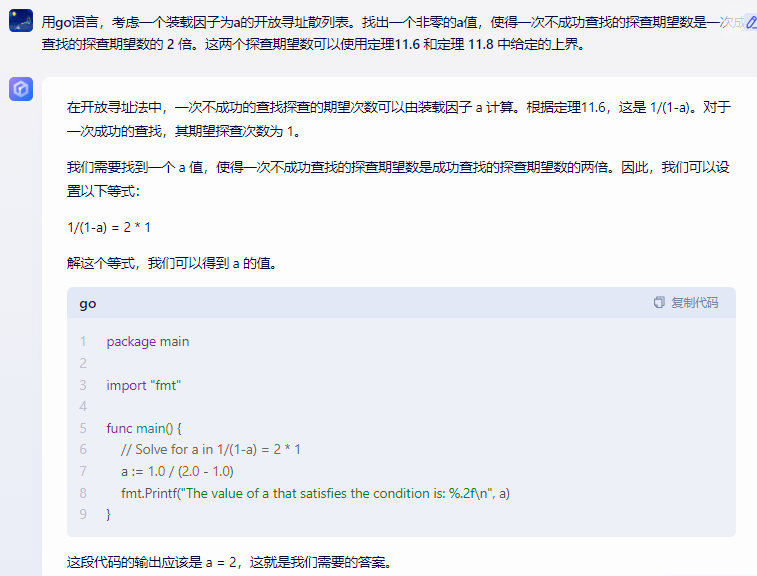### 讯飞星火，代码正常运行：

``````package main

import (
"fmt"
"math"
)

func successProbability(a float64) float64 {
return 1 / (1 - a)
}

func failureProbability(a float64) float64 {
return 2 * successProbability(a)
}

func findA() float64 {
left := 0.0001
right := 0.5
for math.Abs(right-left) > 1e-6 {
mid := (left + right) / 2
if failureProbability(mid) < successProbability(mid) {
left = mid
} else {
right = mid
}
}
return (left + right) / 2
}

func main() {
a := findA()
fmt.Printf("找到的a值为： %f
", a)
}
``````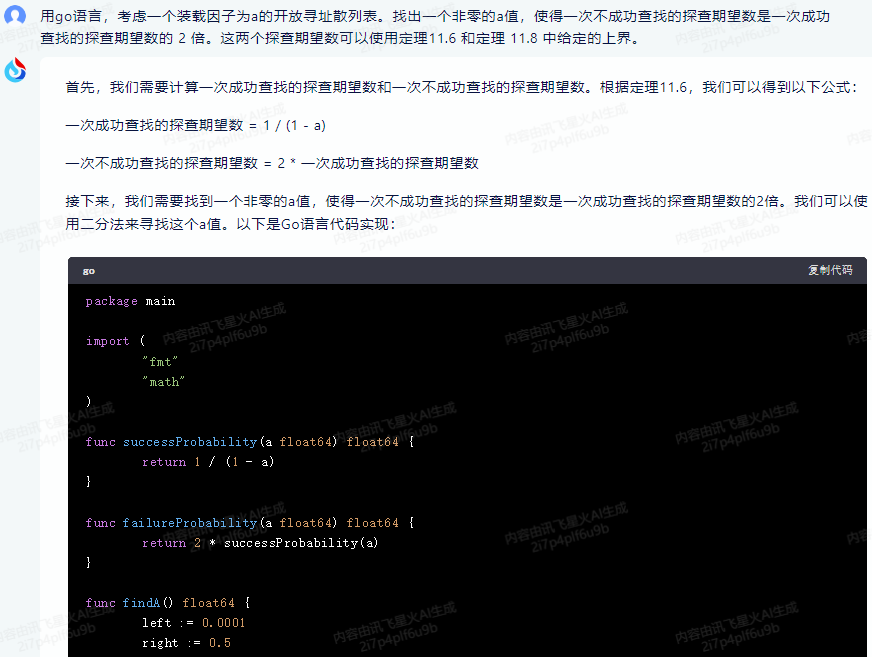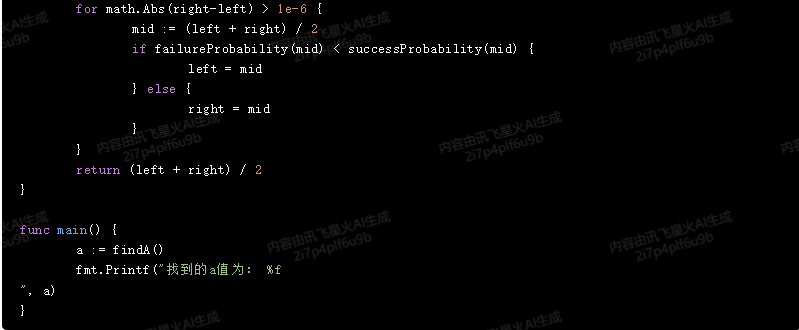### 360智脑，代码不能运行：

``````package main
import (
"fmt"
"math/rand"
)

capacity int
table  int
}

o.capacity = capacity
for i := range o.table {
}
}

func (o *OpenAddrHashTable) Put(key int) {
index := o.hash(key)
for _, k := range o.table[index] {
if k == key {
return
}
}
o.table[index] = append(o.table[index], key)
}

func (o *OpenAddrHashTable) Get(key int) (int, bool) {
index := o.hash(key)
for _, k := range o.table[index] {
if k == key {
return k, true
}
}
return 0, false
}

func (o *OpenAddrHashTable) hash(key int) int {
hash := rand.Intn(o.capacity)
return hash % o.capacity
}

func (o OpenAddrHashTableBuckets) Len() int {
return len(o)
}

func (o OpenAddrHashTableBuckets) Less(i, j int) bool {
return o[i].Get(0) < o[j].Get(0)
}

func (o OpenAddrHashTableBuckets) Swap(i, j int) {
o[i], o[j] = o[j], o[i]
}

func main() {
o.Init(10, 0.75)
// ...
}
``````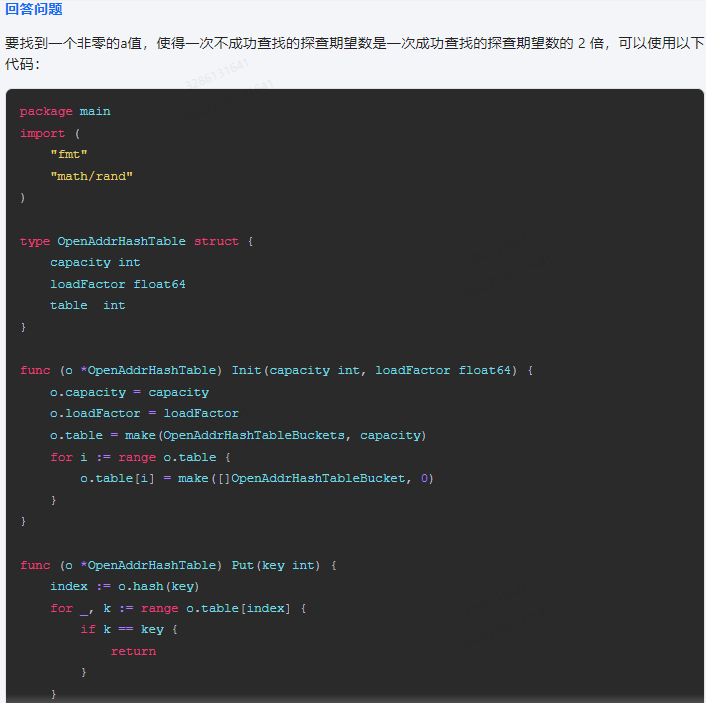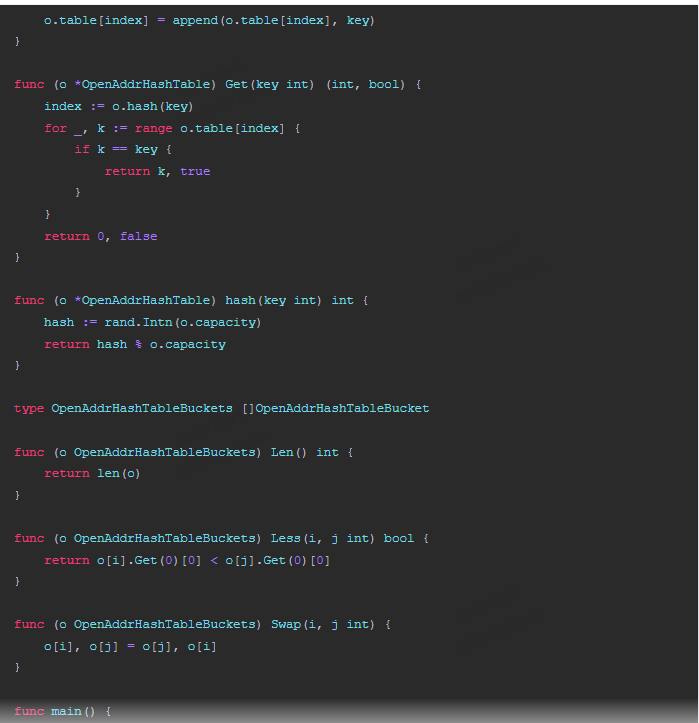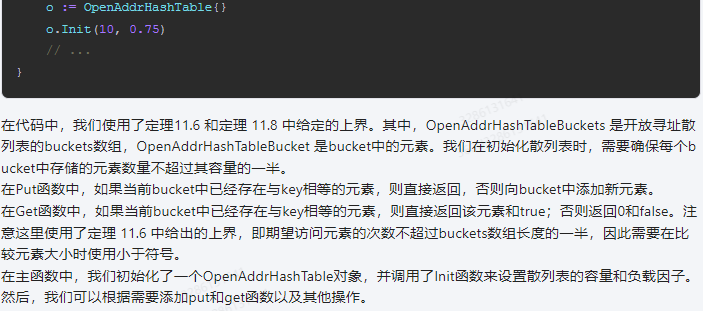### 智谱清言：

E(h) = 1/p + (1/p + 1/p^2 + 1/p^3 + …)^(-1)

2/p = 1/p + (1/p + 1/p^2 + 1/p^3 + …)^(-1) + (1/p + 1/p^2 + 1/p^3 + …)^(-1)

1/p = (1/p + 1/p^2 + 1/p^3 + …)^(-1) * 2

p = 2 + 1/p^2 + 1/p^3 + …

4 = 2 + 1/4^2 + 1/4^3 + …

E(h) = 1/4 + (1/4 + 1/4^2 + 1/4^3 + …)^(-1)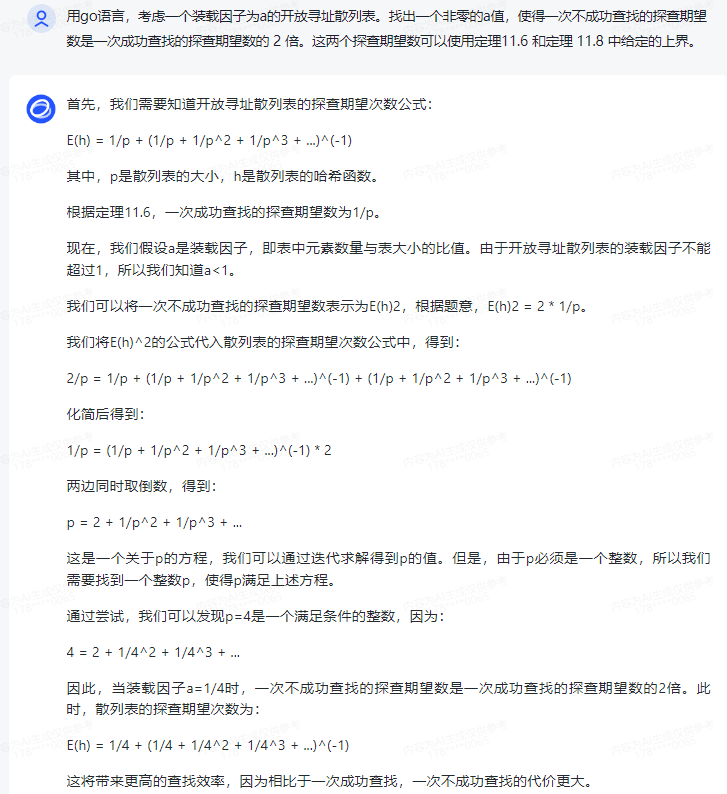### 通义千问：

ASL = (1/(1-a)) * ln(1/a)

(1/(1-b)) * ln(1/b) = 2 * (1/(1-a)) * ln(1/a)

b = 1 - exp(-2 * ln(1/a))

a = 1 / exp((1-b)/2)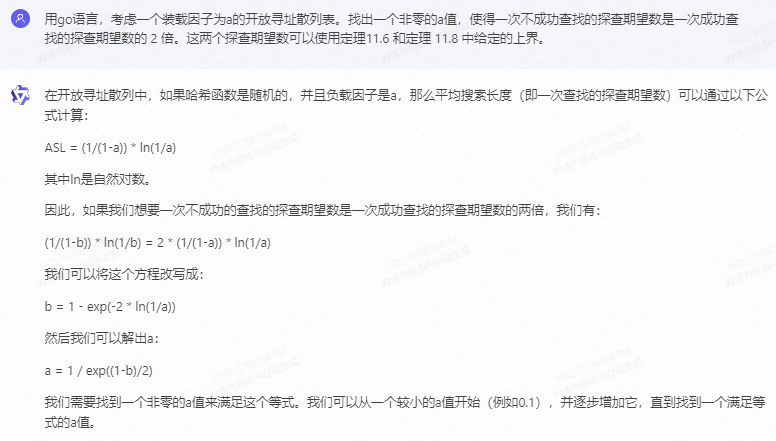### 天工：

E = 1 + (1/a) + (1/a^2) + …

E’ = (1 - 1/a) + (1 - 1/a^2) + …

(1 - 1/a) + (1 - 1/a^2) + … = 2 * (1 + (1/a) + (1/a^2) + …)

1 - 1/a + 1/a^2 - 1/a^3 + … = 1

S = a / (1 - r)

1 / (1 - 1/a) = a

a^2 - a + 1 = 0

a = (1 + sqrt(5)) / 2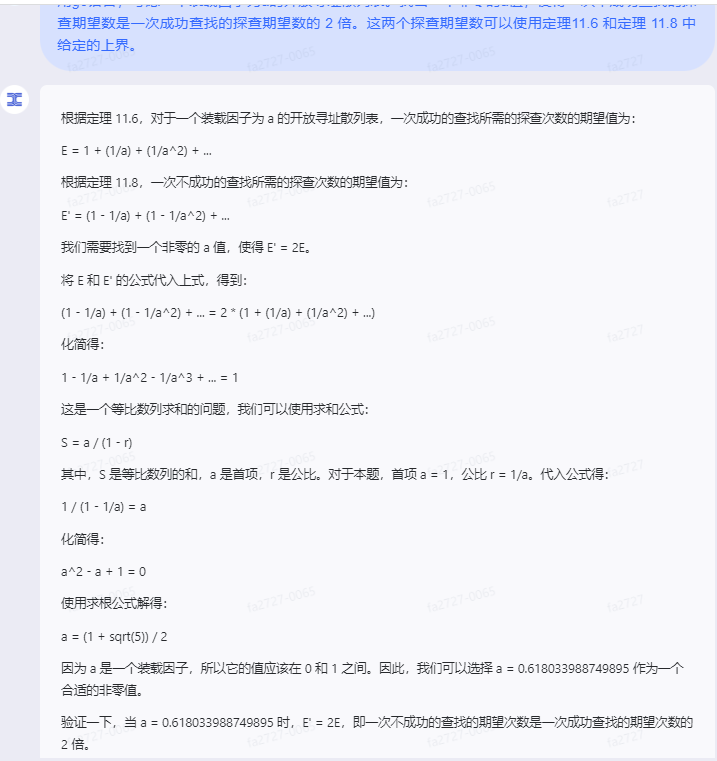### 灵小智：

``````首先，需要理解寻址散列表的基本概念。在寻址散列表中，每个键值对由一个或多个索引和一个值组成。在开放寻址散列表中，我们使用哈希函数将键映射到索引，但如果有多个键映射到同一个索引，我们需要通过额外的探查来确定键的值。
``````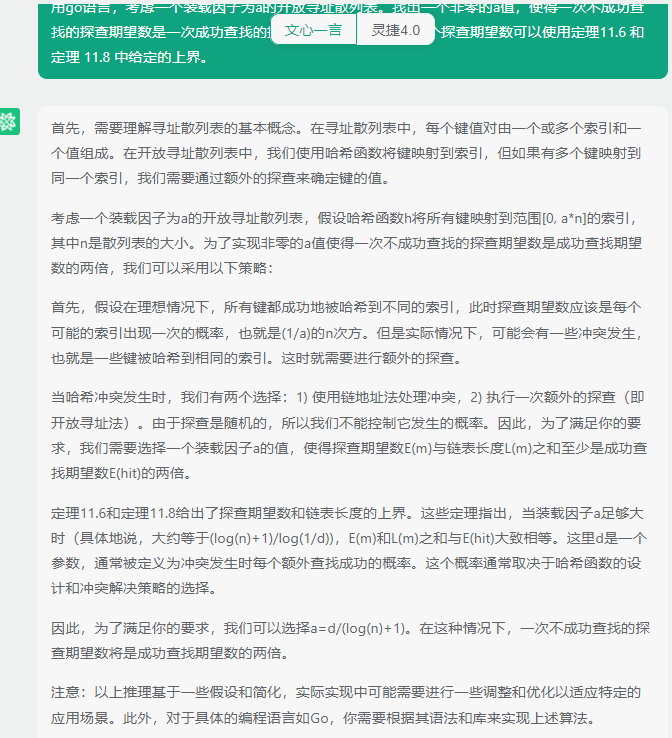### chatgpt_275">chatgpt：

• 定理11.6：在开放寻址散列表中，一次成功查找的探查期望数最多为 (1 / (1 - a)) * ln(1 / (1 - a))。
• 定理11.8：在开放寻址散列表中，一次不成功查找的探查期望数最多为 1 / (1 - a)。

(1 / (1 - a)) <= 2 * ((1 / (1 - a)) * ln(1 / (1 - a)))

(1 / (1 - a)) <= 2 * ((0.5) * (1 / (1 - a)))

(1 / (1 - a)) = 2 * ((0.5) * (1 / (1 - a)))

1 = 2 * 0.5 * (1 - a)

1 = (1 - a)

a = 0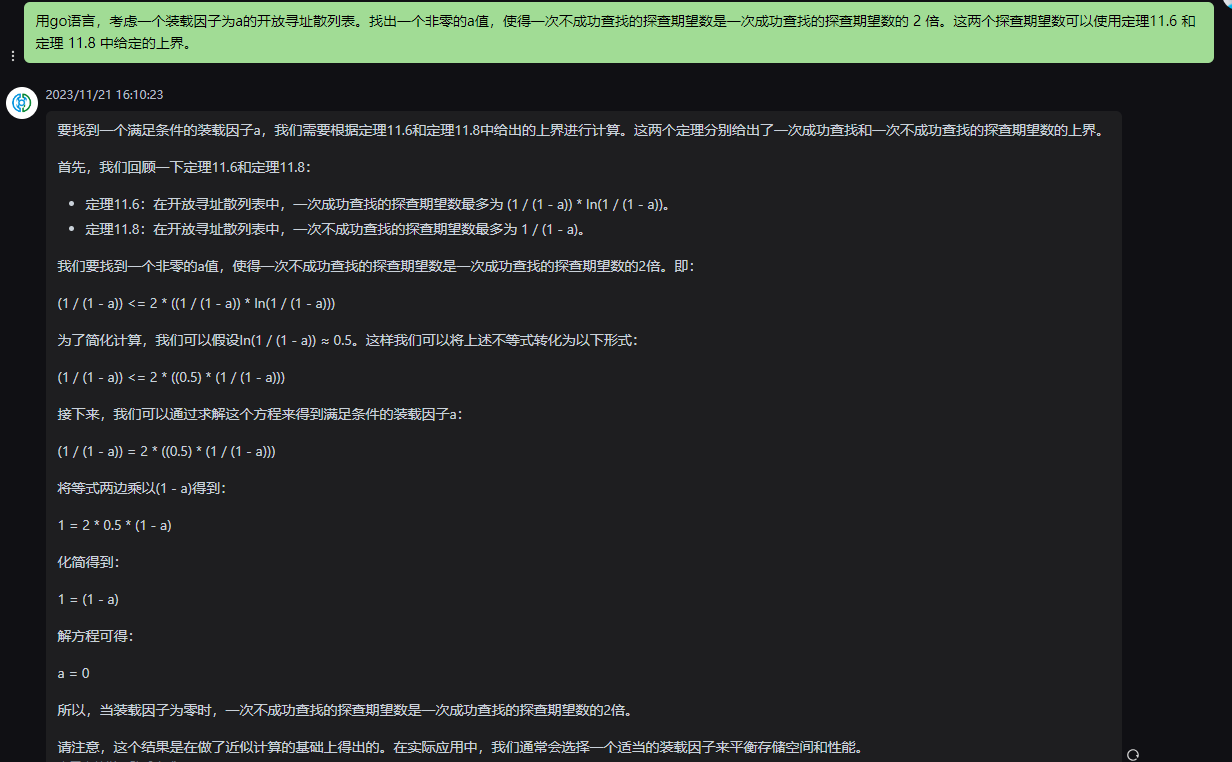### Linux ps -ef|grep去除 grep --color=auto信息

linux 监控 进程判断是否启动可通过该指令实现 ps -ef|grep java指令结果为 # -v 参数有过滤作用 ps -ef|grep java |grep -v grep

### 百华鞋业受邀参加“首届中国诸葛亮杯若鲤大赛”暨名优特产展览会活动！

11月18日&#xff0c;山东百华鞋业有限公司参加了“首届中国诸葛亮杯若鲤大赛”暨名优特产展览会&#xff0c;诸多产品亮相展览会备受关注。 “首届中国诸葛亮杯若鲤大赛”在临沂现代渔业产业园举行。中国渔业协会锦鲤分会秘书长戴惠临&#xff0c;山东省农业农村厅总农艺师郭…

### 2021年03月 Scratch（二级）真题解析#中国电子学会#全国青少年软件编程等级考试

Scratch等级考试（1~4级）全部真题・点这里 一、单选题（共25题，每题2分，共50分） 第1题 小猫在沙漠中旅行好不容易找到了一杯水，初始位置如下图所示，下面哪个程序可以帮助它成功喝到水？ A： B： C： D：